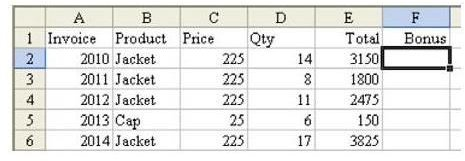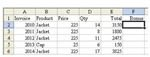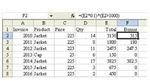## Microsoft Excel: Replace IF Function With Boolean Logic

written by: Mr Excel•edited by: Michele McDonough•updated: 7/6/2011

What if you only need to calculate a formula if the value of a cell satisfies a certain condition? You don't have to use the IF function.

• slide 1 of 2Problem: As shown in Fig. 595, you need to calculate a 10 percent bonus on sales greater than \$1000.00, but no commission is paid on sales less than that amount. While you can use the IF function, is there an easier (and faster) way?

Note: Click any image for a larger view.

Strategy: You can use the Boolean logic facts to do this calculation without an IF function. Remember that a Boolean test that results in a TRUE is treated as a 1 and a FALSE statement is treated as a 0. Thus, you could multiply the calculation E2*0.1 by the Boolean test (E2>1000), as shown in Fig. 596.Summary: This formula combines a math calculation with a Boolean test to produce a valid result. Using these types of calculations is one key to using conditional sums with two conditions. You can learn about these in later tutorials found here at Bright Hub.

Functions Discussed: =IF()

By the way, an IF function would work just as well, too, if you prefer going that route. To learn more about IF constructions, please see How to Use the IF Function in Microsoft Excel 2007. Even though this tutorial was written with Excel 2007 in mind, the technique works in all versions of the spreadsheet software.

• slide 2 of 2

### References and Additional Resources

If you're looking for more tips and tutorials, check out 91 Tips for Calculating With Microsoft Excel. This collection of easy-to-follow guides shows how to customize charts and graphs, different ways to make complex spreadsheets easier to update, various formatting techniques and even how to play games like Craps in Excel.

Still can't find the answer to your question? Leave a note in the Comments section below.

Other Resources:

Microsoft Excel Official Site, http://office.microsoft.com/en-us/excel/

Bill Jelen, Microsoft Excel 2010 In Depth, Available from Amazon.com.